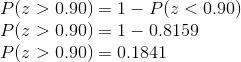# AP Statistics : How to use tables of normal distribution

## Example Questions

### Example Question #1 : How To Use Tables Of Normal Distribution

Find the area under the standard normal curve between Z=1.5 and Z=2.4.

0.0768

.0586

0.3220

0.9000

0.0822

.0586

Explanation: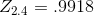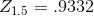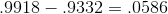### Example Question #2 : How To Use Tables Of Normal Distribution

Alex took a test in physics and scored a 35. The class average was 27 and the standard deviation was 5.

Noah took a chemistry test and scored an 82. The class average was 70 and the standard deviation was 8.

Show that Alex had the better performance by calculating -

1) Alex's standard normal percentile and

2) Noah's standard normal percentile

Alex = .945

Noah = .933

Alex = .778

Noah = .723

Alex = .923

Noah = .911

Alex = .855

Noah = .844

Alex = .901

Noah = .926

Alex = .945

Noah = .933

Explanation:

Alex -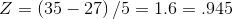on the z-table

Noah -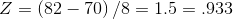on the z-table

### Example Question #3 : Normal Distribution

When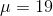and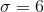Find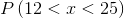.

.72

.68

.76

.61

.81

.72

Explanation: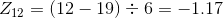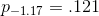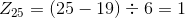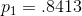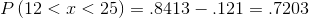### Example Question #4 : Normal Distribution

Arrivals to a bed and breakfast follow a Poisson process. The expected number of arrivals each week is 4. What is the probability that there are exactly 3 arrivals over the course of one week?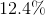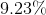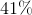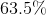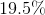Explanation: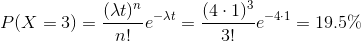### Example Question #5 : Normal Distribution

The masses of tomatoes are normally distributed with a mean ofgrams and a standard deviation ofgrams. What mass of tomatoes would be the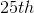percentile of the masses of all the tomatoes?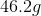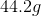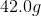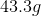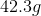Explanation:

The Z score for a normal distribution at thepercentile is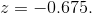So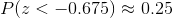, which can be found on the normal distribution table. The mass of tomatoes in thepercentile of all tomatoes  is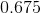standard deviations below the mean, so the mass is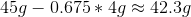.

### Example Question #6 : Normal Distribution

Find.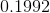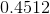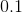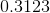Explanation:

First, we use our normal distribution table to find a p-value for a z-score greater than 0.50.

Our table tells us the probability is approximately,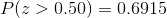.

Next we use our normal distribution table to find a p-value for a z-score greater than 1.23.

Our table tells us the probability is approximately,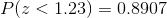.

We then subtract the probability of z being greater than 0.50 from the probability of z being less than 1.23 to give us our answer of,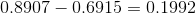.

### Example Question #7 : Normal Distribution

Find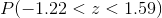.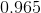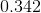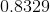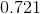Explanation:

First, we use the table to look up a p-value for z > -1.22.

This gives us a p-value of,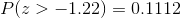.

Next, we use the table to look up a p-value for z > 1.59.

This gives us a p-value of,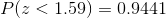.

Finally we subtract the probability of z being greater than -1.22 from the probability of z being less than 1.59 to arrive at our answer of,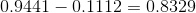.

### Example Question #8 : Normal Distribution

Gabbie earned a score of 940 on a national achievement test. The mean test score was 850 with a sample standard deviation of 100. What proportion of students had a higher score than Gabbie? (Assume that test scores are normally distributed.)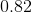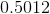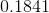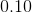Explanation:

When we get this type of problem, first we need to calculate a z-score that we can use in our table.

To do that, we use our z-score formula: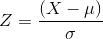where,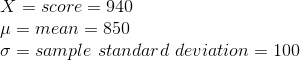Plugging into the equation we get: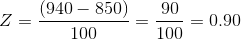We then use our table to look up a p-value for z > 0.9. Since we want to calculate the probability of students who earned a higher score than Gabbie we need to subtract the P(z<0.9) to get our answer.